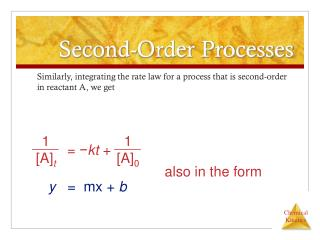DownloadDownload PresentationSecond-Order Processes

# Second-Order Processes

Télécharger la présentation## Second-Order Processes

- - - - - - - - - - - - - - - - - - - - - - - - - - - E N D - - - - - - - - - - - - - - - - - - - - - - - - - - -
##### Presentation Transcript

1. 1 [A]0 1 [A]t = −kt + Second-Order Processes Similarly, integrating the rate law for a process that is second-order in reactant A, we get also in the form y = mx + b

2. 1 [A]0 1 [A]t = −kt + Second-Order Processes So if a process is second-order in A, a plot of 1/[A] vs. t will yield a straight line, and the slope of that line is k.

3. NO2(g) NO (g) + 1/2 O2(g) Second-Order Processes The decomposition of NO2 at 300°C is described by the equation and yields data comparable to this:

4. Second-Order Processes • Graphing ln [NO2] vs.t yields: • The plot is not a straight line, so the process is not first-order in [A].

5. Second-Order Processes • Graphing ln 1/[NO2] vs. t, however, gives this plot. • Because this is a straight line, the process is second-order in [A].

6. Half-Life • Half-life is defined as the time required for one-half of a reactant to react. • Because [A] at t1/2 is one-half of the original [A], [A]t = 0.5 [A]0.

7. 0.5 [A]0 [A]0 ln = −kt1/2 0.693 k = t1/2 Half-Life For a first-order process, this becomes ln 0.5 = −kt1/2 −0.693 = −kt1/2 NOTE: For a first-order process, the half-life does not depend on [A]0.

8. 1 k[A]0 1 [A]0 1 [A]0 1 [A]0 2 [A]0 1 0.5 [A]0 2 − 1 [A]0 = kt1/2 + = kt1/2 + = = kt1/2 = t1/2 Half-Life For a second-order process,

9. Temperature and Rate • Generally, as temperature increases, so does the reaction rate. • This is because k is temperature dependent.

10. The Collision Model • In a chemical reaction, bonds are broken and new bonds are formed. • Molecules can only react if they collide with each other.

11. The Collision Model Furthermore, molecules must collide with the correct orientation and with enough energy to cause bond breakage and formation.

12. Activation Energy • In other words, there is a minimum amount of energy required for reaction: the activation energy, Ea. • Just as a ball cannot get over a hill if it does not roll up the hill with enough energy, a reaction cannot occur unless the molecules possess sufficient energy to get over the activation energy barrier.

13. Reaction Coordinate Diagrams It is helpful to visualize energy changes throughout a process on a reaction coordinate diagram like this one for the rearrangement of methyl isonitrile.

14. Reaction Coordinate Diagrams • It shows the energy of the reactants and products (and, therefore, E). • The high point on the diagram is the transition state. • The species present at the transition state is called the activated complex. • The energy gap between the reactants and the activated complex is the activation energy barrier.

15. Maxwell–Boltzmann Distributions • Temperature is defined as a measure of the average kinetic energy of the molecules in a sample. • At any temperature there is a wide distribution of kinetic energies.

16. Maxwell–Boltzmann Distributions • As the temperature increases, the curve flattens and broadens. • Thus at higher temperatures, a larger population of molecules has higher energy.

17. Maxwell–Boltzmann Distributions • If the dotted line represents the activation energy, as the temperature increases, so does the fraction of molecules that can overcome the activation energy barrier. • As a result, the reaction rate increases.

18. Maxwell–Boltzmann Distributions This fraction of molecules can be found through the expression where R is the gas constant and T is the Kelvin temperature. f = e−Ea/RT

19. Arrhenius Equation Svante Arrhenius developed a mathematical relationship between k and Ea: k = A e−Ea/RT where A is the frequency factor, a number that represents the likelihood that collisions would occur with the proper orientation for reaction.

20. 1 RT Arrhenius Equation Taking the natural logarithm of both sides, the equation becomes ln k = -Ea() + ln A y = mx + b Therefore, if k is determined experimentally at several temperatures, Eacan be calculated from the slope of a plot of ln k vs. 1/T.

21. Reaction Mechanisms The sequence of events that describes the actual process by which reactants become products is called the reaction mechanism.

22. Reaction Mechanisms • Reactions may occur all at once or through several discrete steps. • Each of these processes is known as an elementary reaction or elementary process.

23. Reaction Mechanisms The molecularity of a process tells how many molecules are involved in the process.

24. Multistep Mechanisms • In a multistep process, one of the steps will be slower than all others. • The overall reaction cannot occur faster than this slowest, rate-determining step.

25. Slow Initial Step NO2(g) + CO (g) NO (g) + CO2(g) • The rate law for this reaction is found experimentally to be Rate = k [NO2]2 • CO is necessary for this reaction to occur, but the rate of the reaction does not depend on its concentration. • This suggests the reaction occurs in two steps.

26. Slow Initial Step • A proposed mechanism for this reaction is Step 1: NO2 + NO2 NO3 + NO (slow) Step 2: NO3 + CO  NO2 + CO2 (fast) • The NO3 intermediate is consumed in the second step. • As CO is not involved in the slow, rate-determining step, it does not appear in the rate law.